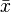# Blog Archives

## MEAN

In statistics, mean has two related meanings:

• the arithmetic mean (and is distinguished from the geometric mean or harmonic mean).
• the expected value of a random variable, which is also called the population mean.

There are other statistical measures that use samples that some people confuse with averages – including ‘median’ and ‘mode’. Other simple statistical analyses use measures of spread, such as range, interquartile range, or standard deviation. For a real-valued random variable X, the mean is the expectation of X. Note that not every probability distribution has a defined mean (or variance); see the Cauchy distribution for an example.

For a data set, the mean is the sum of the values divided by the number of values. The mean of a set of numbers x1, x2, …, xn is typically denoted by, pronounced “x bar”. This mean is a type of arithmetic mean. If the data set were based on a series of observations obtained by sampling a statistical population, this mean is termed the “sample mean” () to distinguish it from the “population mean” (μ or μx). The mean is often quoted along with the standard deviation: the mean describes the central location of the data, and the standard deviation describes the spread. An alternative measure of dispersion is the mean deviation, equivalent to the average absolute deviation from the mean. It is less sensitive to outliers, but less mathematically tractable.